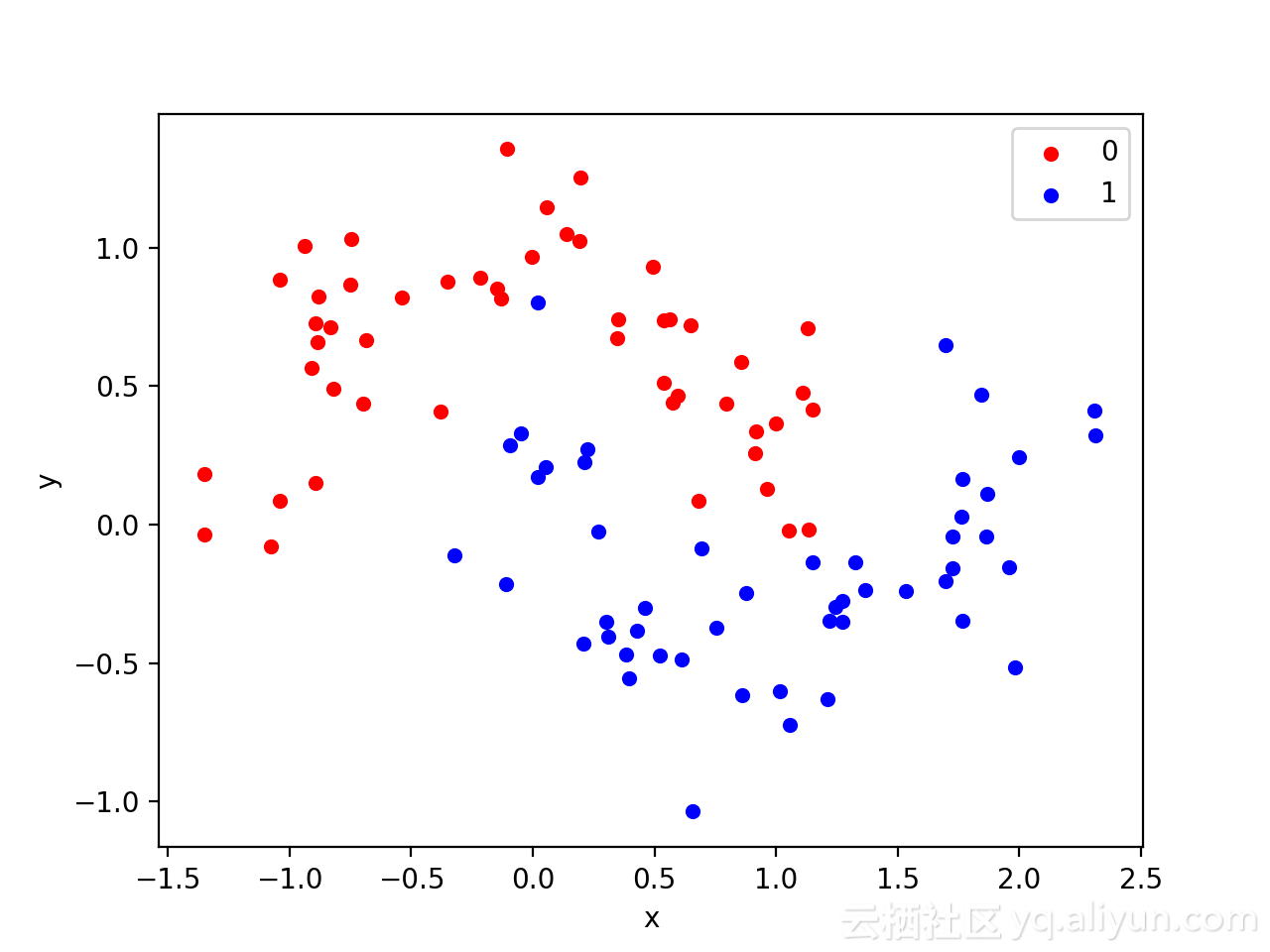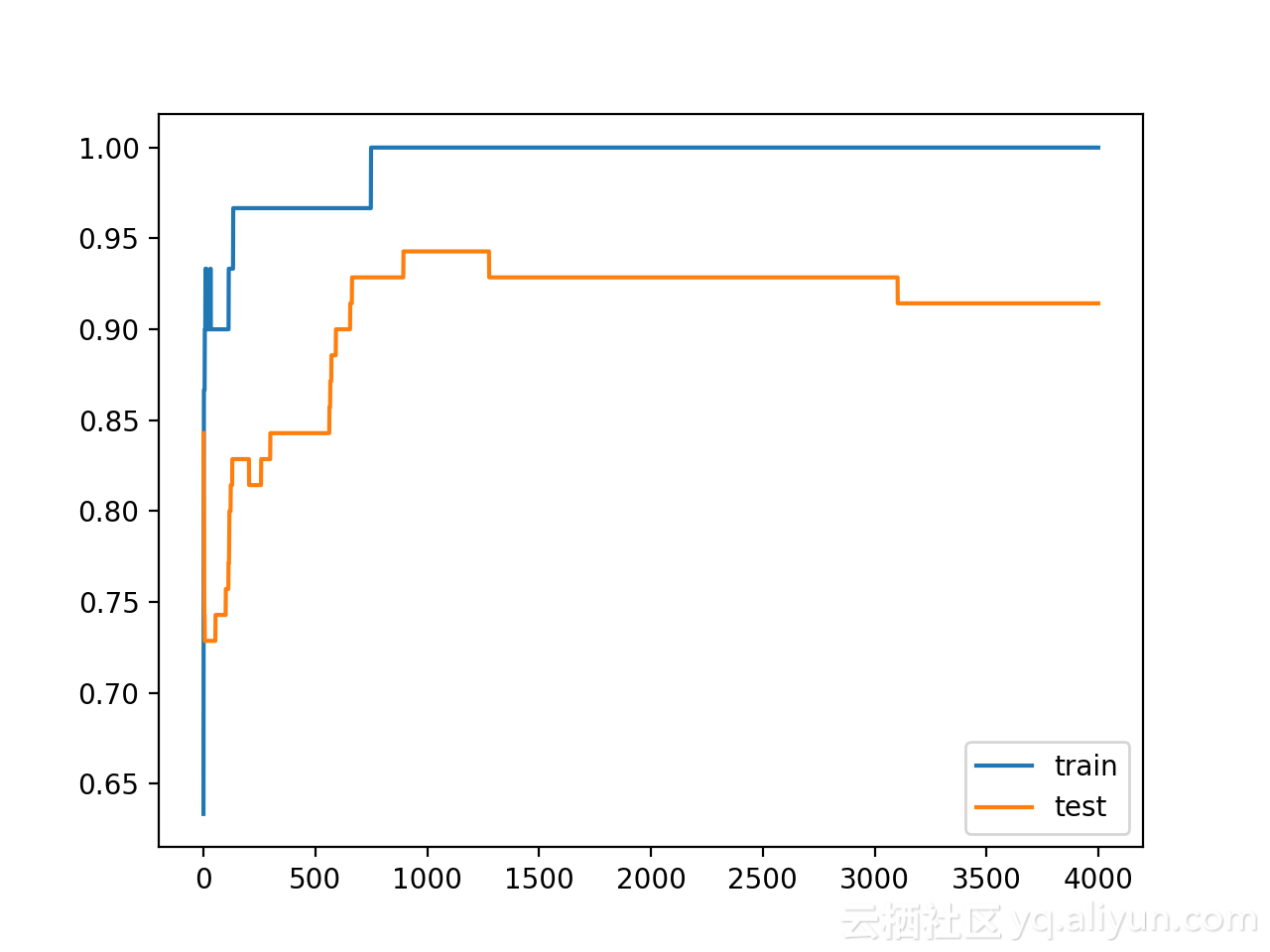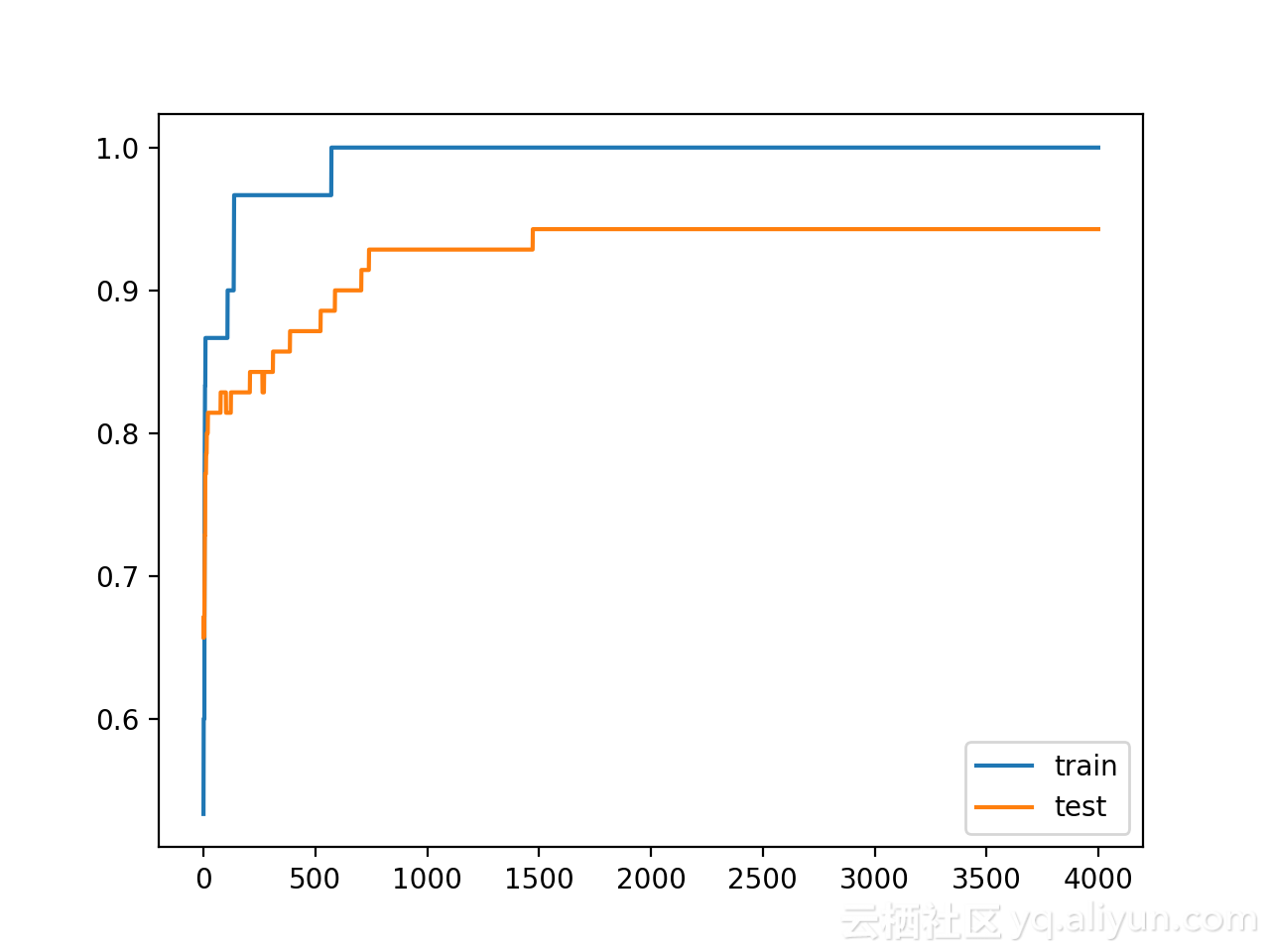深度学习Trick——用权重约束减轻深层网络过拟合|附(Keras)实现代码

+关注继续查看

在深度学习中，批量归一化（batch normalization）以及对损失函数加一些正则项这两类方法，一般可以提升模型的性能。这两类方法基本上都属于权重约束，用于减少深度学习神经网络模型对训练数据的过拟合，并改善模型对新数据的性能。
目前，存在多种类型的权重约束方法，例如最大化或单位向量归一化，有些方法也必须需要配置超参数。
在本教程中，使用Keras API，用于向深度学习神经网络模型添加权重约束以减少过拟合。
完成本教程后，您将了解：

• 如何使用Keras API创建向量范数约束；
• 如何使用Keras API为MLP、CNN和RNN层添加权重约束；
• 如何通过向现有模型添加权重约束来减少过度拟合；• Keras中的权重约束；
• 图层上的权重约束；
• 权重约束案例研究；

Keras中权重约束

Keras API支持权重约束，且约束可以按每层指定。
使用约束通常涉及在图层上为输入权重设置kernel_constraint参数，偏差权重设置为bias_constraint。通常，权重约束方法不涉及偏差权重。
一组不同的向量规范在keras.constraints模块可以用作约束：

• 最大范数（max_norm）：强制权重等于或低于给定限制;
• 非负规范（non_neg）：强制权重为正数；
• 单位范数（unit_norm）：强制权重为1.0；
• Min-Max范数（min_max_norm）：强制权重在一个范围之间；

# import norm
from keras.constraints import max_norm
# instantiate norm
norm = max_norm(3.0)

图层上的权重约束

权重规范可用于Keras的大多数层，下面介绍一些常见的例子：

MLP权重约束

下面的示例是在全连接层上设置最大范数权重约束：

# example of max norm on a dense layer
from keras.layers import Dense
from keras.constraints import max_norm
...
...

CNN权重约束

# example of max norm on a cnn layer
from keras.layers import Conv2D
from keras.constraints import max_norm
...
...

RNN权重约束

与其他图层类型不同，递归神经网络允许我们对输入权重和偏差以及循环输入权重设置权重约束。通过图层的recurrent_constraint参数设置递归权重的约束。
下面的示例是在LSTM图层上设置最大范数权重约束：

# example of max norm on an lstm layer
from keras.layers import LSTM
from keras.constraints import max_norm
...
...

基于以上的基本知识，下面进行实例实践。

权重约束案例研究

在本节中，将演示如何使用权重约束来减少MLP对简单二元分类问题的过拟合问题。
此示例只是提供了一个模板，读者可以举一反三，将权重约束应用于自己的神经网络以进行分类和回归问题。

二分类问题

使用标准二进制分类问题来定义两个半圆观察，每个类一个半圆。其中，每个观测值都有两个输入变量，它们具有相同的比例，输出值分别为0或1，该数据集也被称为“ 月亮”数据集，这是由于绘制时，每个类中出现组成的形状类似于月亮。
可以使用make_moons()函数生成观察结果，设置参数为添加噪声、随机关闭，以便每次运行代码时生成相同的样本。

# generate 2d classification dataset
X, y = make_moons(n_samples=100, noise=0.2, random_state=1)

可以在图表上绘制两个变量x和y坐标，并将数据点所属的类别的颜色作为观察的颜色。
下面列出生成数据集并绘制数据集的完整示例：

# generate two moons dataset
from sklearn.datasets import make_moons
from matplotlib import pyplot
from pandas import DataFrame
# generate 2d classification dataset
X, y = make_moons(n_samples=100, noise=0.2, random_state=1)
# scatter plot, dots colored by class value
df = DataFrame(dict(x=X[:,0], y=X[:,1], label=y))
colors = {0:'red', 1:'blue'}
fig, ax = pyplot.subplots()
grouped = df.groupby('label')
for key, group in grouped:
group.plot(ax=ax, kind='scatter', x='x', y='y', label=key, color=colors[key])
pyplot.show()

运行该示例会创建一个散点图，可以从图中看到，对应类别显示的图像类似于半圆形或月亮形状。上图的数据集表明它是一个很好的测试问题，因为不能用直线划分，需要非线性方法，比如神经网络来解决。
只生成了100个样本，这对于神经网络而言较小，也提供了过拟合训练数据集的概率，并且在测试数据集上具有更高的误差。因此，也是应用正则化的一个好例子。此外，样本具有噪声，使模型有机会学习不一致的样本的各个方面。

多层感知器过拟合

在机器学习力，MLP模型可以解决这类二进制分类问题。
MLP模型只具有一个隐藏层，但具有比解决该问题所需的节点更多的节点，从而提供过拟合的可能。
在定义模型之前，需要将数据集拆分为训练集和测试集，按照3:7的比例将数据集划分为训练集和测试集。

# generate 2d classification dataset
X, y = make_moons(n_samples=100, noise=0.2, random_state=1)
# split into train and test
n_train = 30
trainX, testX = X[:n_train, :], X[n_train:, :]
trainy, testy = y[:n_train], y[n_train:]

接下来，定义模型。隐藏层的节点数设置为500、激活函数为RELU，但在输出层中使用Sigmoid激活函数以预测输出类别为0或1。

# define model
model = Sequential()

然后，设置迭代次数为4,000次，默认批量训练样本数量为32。

# fit model
history = model.fit(trainX, trainy, validation_data=(testX, testy), epochs=4000, verbose=0)

这里将测试数据集作为验证数据集验证算法的性能：

# evaluate the model
_, train_acc = model.evaluate(trainX, trainy, verbose=0)
_, test_acc = model.evaluate(testX, testy, verbose=0)
print('Train: %.3f, Test: %.3f' % (train_acc, test_acc))

最后，绘制出模型每个时期在训练和测试集上性能。如果模型确实对训练数据集过拟合了，对应绘制的曲线将会看到，模型在训练集上的准确度继续增加，而测试集上的性能是先上升，之后下降。

# plot history
pyplot.plot(history.history['acc'], label='train')
pyplot.plot(history.history['val_acc'], label='test')
pyplot.legend()
pyplot.show()

将以上过程组合在一起，列出完整示例：

# mlp overfit on the moons dataset
from sklearn.datasets import make_moons
from keras.layers import Dense
from keras.models import Sequential
from matplotlib import pyplot
# generate 2d classification dataset
X, y = make_moons(n_samples=100, noise=0.2, random_state=1)
# split into train and test
n_train = 30
trainX, testX = X[:n_train, :], X[n_train:, :]
trainy, testy = y[:n_train], y[n_train:]
# define model
model = Sequential()
# fit model
history = model.fit(trainX, trainy, validation_data=(testX, testy), epochs=4000, verbose=0)
# evaluate the model
_, train_acc = model.evaluate(trainX, trainy, verbose=0)
_, test_acc = model.evaluate(testX, testy, verbose=0)
print('Train: %.3f, Test: %.3f' % (train_acc, test_acc))
# plot history
pyplot.plot(history.history['acc'], label='train')
pyplot.plot(history.history['val_acc'], label='test')
pyplot.legend()
pyplot.show()

运行该示例，给出模型在训练数据集和测试数据集上的性能。
可以看到模型在训练数据集上的性能优于测试数据集，这是发生过拟合的标志。鉴于神经网络和训练算法的随机性，每次仿真的具体结果可能会有所不同。因为模型是过拟合的，所以通常不会期望在相同数据集上能够重复运行得到相同的精度。

Train: 1.000, Test: 0.914

创建一个图，显示训练和测试集上模型精度的线图。从图中可以看到模型过拟合时的预期形状，其中测试精度达到一个临界点后再次开始减小。具有权重约束的多层感知器过拟合

为了和上面做对比，现在对MLP使用权重约束。目前，有一些不同的权重约束方法可供选择。本文选用一个简单且好用的约束——简单地标准化权重，使得其范数等于1.0，此约束具有强制所有传入权重变小的效果。
在Keras中可以通过使用unit_norm来实现，并且将此约束添加到第一个隐藏层，如下所示：

此外，也可以通过使用min_max_norm并将minmaximum设置为1.0 来实现相同的结果，例如：

但是无法通过最大范数约束获得相同的结果，因为它允许规范等于或低于指定的限制; 例如：

下面列出具有单位规范约束的完整代码：

# mlp overfit on the moons dataset with a unit norm constraint
from sklearn.datasets import make_moons
from keras.layers import Dense
from keras.models import Sequential
from keras.constraints import unit_norm
from matplotlib import pyplot
# generate 2d classification dataset
X, y = make_moons(n_samples=100, noise=0.2, random_state=1)
# split into train and test
n_train = 30
trainX, testX = X[:n_train, :], X[n_train:, :]
trainy, testy = y[:n_train], y[n_train:]
# define model
model = Sequential()
# fit model
history = model.fit(trainX, trainy, validation_data=(testX, testy), epochs=4000, verbose=0)
# evaluate the model
_, train_acc = model.evaluate(trainX, trainy, verbose=0)
_, test_acc = model.evaluate(testX, testy, verbose=0)
print('Train: %.3f, Test: %.3f' % (train_acc, test_acc))
# plot history
pyplot.plot(history.history['acc'], label='train')
pyplot.plot(history.history['val_acc'], label='test')
pyplot.legend()
pyplot.show()

运行该示例，给出模型在训练数据集和测试数据集上的性能。
从下图可以看到，对权重进行严格约束确实提高了模型在验证集上的性能，并且不会影响训练集的性能。

Train: 1.000, Test: 0.943

从训练和测试精度曲线图来看，模型已经在训练数据集上不再过拟合了，且模型在训练和测试数据集的精度保持在一个稳定的水平。扩展

• 报告权重标准：更新示例以计算网络权重的大小，并证明使用约束后，确实使得幅度更小；
• 约束输出层：更新示例以将约束添加到模型的输出层并比较结果；
• 约束偏置：更新示例以向偏差权重添加约束并比较结果；
• 反复评估：更新示例以多次拟合和评估模型，并报告模型性能的均值和标准差；

进一步阅读

如果想进一步深入了解，下面提供一些有关该主题的其它资源：

作者信息

Jason Brownlee，机器学习专家1. winlin winlinvip simple-rtmp-server  https://github.com/winlinvip/simple-rtmp-server SRS定位是运营级的互联网直播服务器集群，追求更好的概念完整性和最简单实现的代码。
1332 0Keras搭建深度神经网络解决多分类问题
2489 03132 01460 06544 0Android EditText聚焦时hint消失的简单代码
999 01769 0git学习------&gt;从SVN迁移到Git之后，项目开发代码继续在SVN提交，如何同步迁移之后继续在SVN提交的代码到Git？

1596 02911 03084 0
【方向】

707

5

《2021云上架构与运维峰会演讲合集》

《零基础CSS入门教程》

《零基础HTML入门教程》